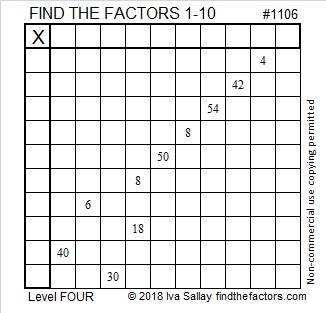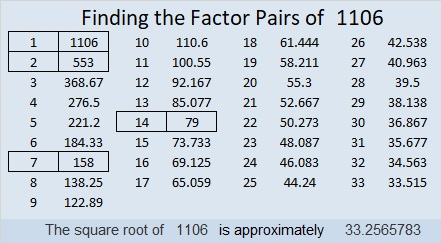# 1106 and Level 4

Can you use logic to figure out where all the numbers from 1 to 10 need to go in both the first column and the top row so that this puzzle can become a multiplication table? Give it a try. It’s fun!Print the puzzles or type the solution in this excel file: 10-factors-1102-1110

Here is some information about the number 1106:

• 1106 is a composite number.
• Prime factorization: 1106 = 2 × 7 × 79
• The exponents in the prime factorization are 1, 1, and 1. Adding one to each and multiplying we get (1 + 1)(1 + 1)(1 + 1) = 2 × 2 × 2 = 8. Therefore 1106 has exactly 8 factors.
• Factors of 1106: 1, 2, 7, 14, 79, 158, 553, 1106
• Factor pairs: 1106 = 1 × 1106, 2 × 553, 7 × 158, or 14 × 79
• 1106 has no square factors that allow its square root to be simplified. √1106 ≈ 33.256581106 is repdigit 222 in BASE 23 because 2(23² + 23+ 1) = 2(553) = 1106